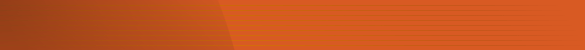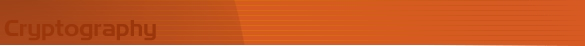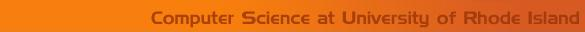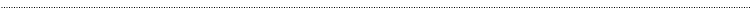Home Page Introduction Classical Ciphers Enigma Machine DES Key Agreement PGP R.S.A. Number Theory Other LinksEuclid's AlgorithmEuclid's algorithm is used for finding the greatest common denominator of two integers. The greatest common denominator of two integers is defined as the largest integer that divides both numbers, without a remainder. All pairs of numbers have at least the number 1 as a common divisor, and sometimes 1 is the greatest common divisor for a given pair. Such numbers are said to be relatively prime (or coprime) to each other. Greatest common divisors are used in reducing fractions, polynomial manipulations, factorization, integration and more.

The equation for finding the greatest common denominators is:

gcd(m,n) = m, if n = 0

gcd(m,n) = gcd(n, m mod n), if n is not 0

Suppose we wanted to find the greatest common denominator of the two integers 1674 and 498. We would computer gcd(1674, 498). First we check that n, 498, is not zero, so we need to compute m mod n. 1674/498 = 3, with a remainder of 180, so now we compute gcd(498, 180). Notice that the positions of the number 498 switches (we went from gcd(1674, 498) to gcd(498, 180)).

We continue this process until n = 0. 498 mod 180 = 138, so next we will compute gcd(180, 138). Then, we compute 180 mod 138 = 42, so we will do gcd(138, 42). 138 mod 42 = 12, so gcd(42, 12). Then, 42 mod 12 = 6, so gcd(12, 6). Next, 12 mod 6 = 0, so our last step will be to compute gcd(6, 0). Now, n = 0, so the greatest common divisor is m = 6. The greatest common divisor of 1674 and 498 is 6.